Checkout JEE MAINS 2022 Question Paper Analysis : Checkout JEE MAINS 2022 Question Paper Analysis :

# Lines and Angles Introduction

Geometry is composed of two ancient Greek words: Geo and Metron. Geo means Earth, and Metron means measurement. Geometry is the branch of mathematics that deals with shape, size, position, spatial relationships and properties of different figures. Various parameters are involved in defining a shape, and the essential parameters are lines and angles. In this article, we are going to discuss two important parameters called lines and angles in detail.

## Definition of Lines and Angles

The entire geometry begins with a point. A point is a dimensionless entity that specifies the location or position, and it is represented using a dot symbol, and its length is zero.

### What are Lines?

All the shapes that we see around us consist of an infinite number of points. When a point moves so that its direction remains unaltered, then a straight line is obtained. In other words, a one-dimensional collection of points extending infinitely in both directions represents a line, as shown below. A line never ends.

Line

A line is a one dimensional figure with a straight set of points that extend in opposite directions. That means, it has no ends in both directions(infinite) and has no thickness.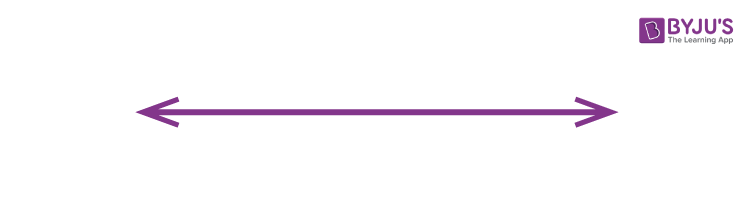Line Segment

A lines segment is a portion of a line with two endpoints as shown in the figure given below: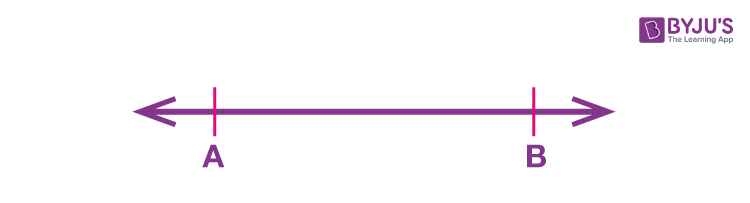Ray:

A ray is a part of a line that has one endpoint (i.e. starting point) and it extends in one direction infinitely.Apart from the above, there are different types of lines such as parallel, perpendicular, intersecting and non-intersecting lines, etc.

 Parallel lines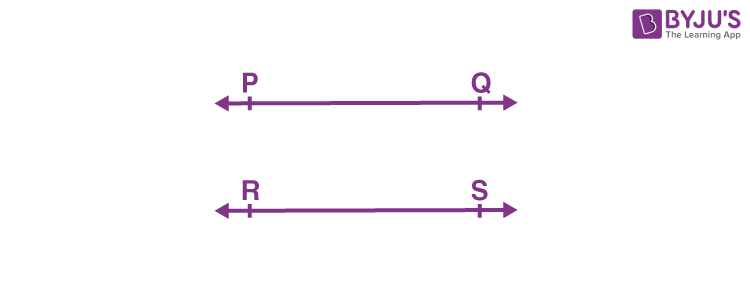Perpendicular lines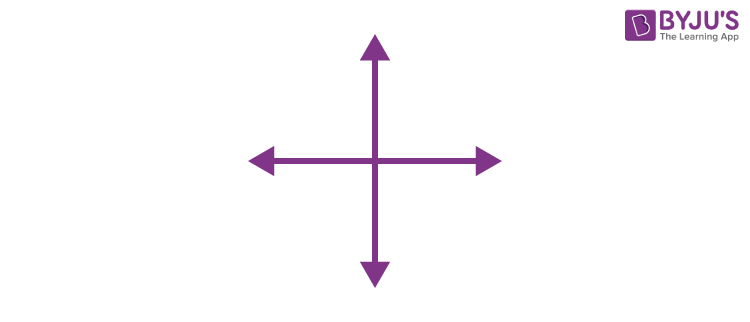Intersecting lines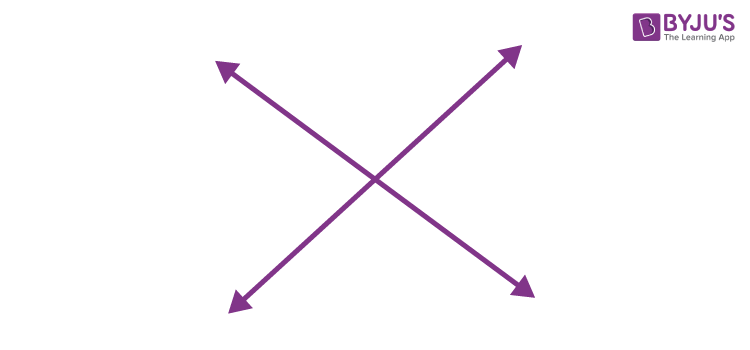Non-intersecting lines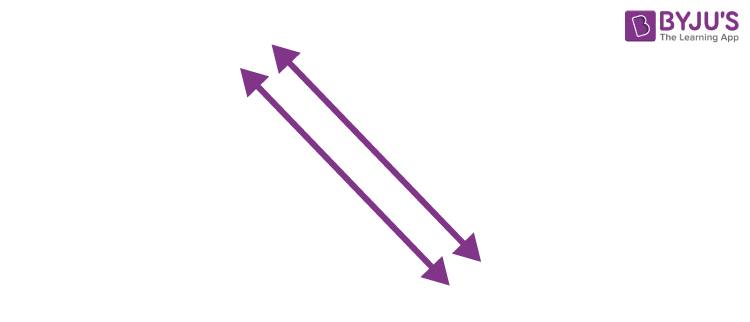### What is an Angle?

If a ray is rotated about its endpoint then the measure of its rotation between the final and initial position of the ray is known as an angle. In fig. 3,

$$\begin{array}{l}\overrightarrow{ST}\end{array}$$
is the initial position of the ray and when it is rotated about its endpoint i.e. S, the final position is represented by ray
$$\begin{array}{l}\overrightarrow{SR}\end{array}$$
. The measure of this rotation is measured in angles. The angle between the initial and final position of a ray is measured as ∠RST.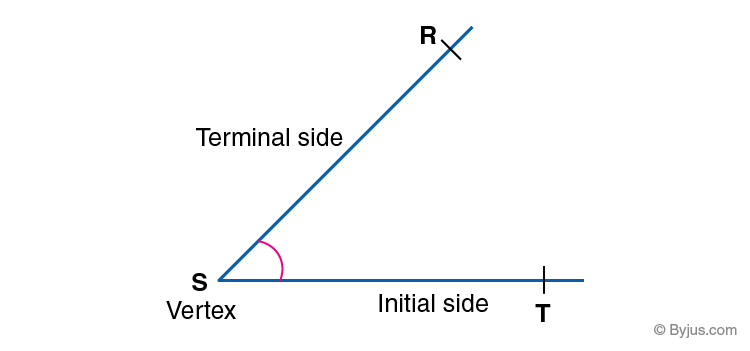Also, check: Angles

Angles are classified into different types based on the measure of angle between the initial and terminal side. They are:

Acute angles: Less than 90 degrees

Right angle: Equal to 90 degrees

Obtuse angle: Greater than 90 degrees and less than 180 degrees

Straight angle: Equal to 180 degrees

Reflex angle: Greater than 180 degrees and less than 360 degrees

Full angle or Complete angle: Equal to 360 degrees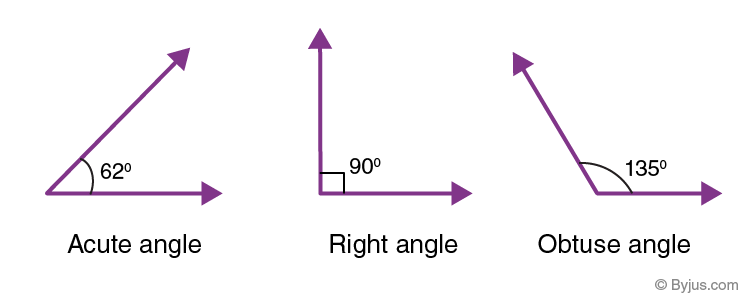To solve more problems on topic Lines and Angles visit BYJU’S – The Learning App, which provides detailed and step by step solutions to all questions in an NCERT Books. Also, take free tests to practice for exams.

## Video Lesson on Types of Angles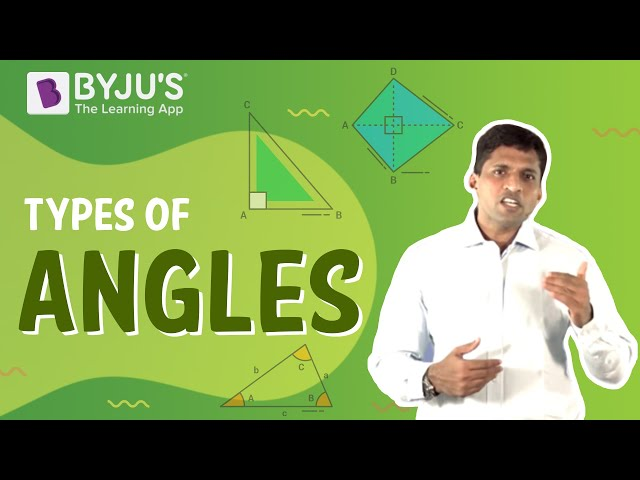Quiz on Introduction to lines and angles

#### 1 Comment

1. Vijeta S H

🙏🏻was helpful. Great job. Prepare more and more quotes like this and make level bit hard.